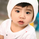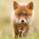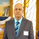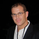2079 views
2079
Request for DCC/coondawg71
added support for using session.

theres a issue with the session it needs to have the format inverted:
(start-end) to (end-start)
'0000-1500' to '1500-0000'
//@version=2
study(title='[RS]Open Range Breakout V3', shorttitle='ORB', overlay=true)
//  Request for DCC
mode = input(title='Mode (1:timeframe, 2:session):', type=integer, minval=1, maxval=2, defval=1)
tf = input(title='Timeframe for open range:', type=string, defval='60', confirm=false)
tf2 = input(title='Timeframe for range capture:', type=string, defval='D', confirm=false)
sess = input(title='Session for mode 2:', type=string, defval='0400-1500')

f_is_new_day(_mode) => _mode == 1 ? change(time(tf2))!=0 : _mode == 2 ? change(time(tf2, sess))!=0 : false

ND_open = f_is_new_day(mode) ? security(tickerid, tf, open) : ND_open
ND_high = f_is_new_day(mode) ? security(tickerid, tf, high) : ND_high
ND_low = f_is_new_day(mode) ? security(tickerid, tf, low) : ND_low

ND_stretch = na(ND_stretch) ? 0 : f_is_new_day(mode) ? (ND_stretch*9 + security(tickerid, tf, (high-open)>=(open-low)?high-open:open-low)) / 10 : ND_stretch

filter_high = f_is_new_day(mode) ? na : ND_high
filter_low = f_is_new_day(mode) ? na : ND_low

filter_high_stretch = f_is_new_day(mode) ? na : ND_high+ND_stretch
filter_low_stretch = f_is_new_day(mode) ? na : ND_low-ND_stretch

fh = plot(title='TR', series=filter_high, style=linebr, color=black)
fl = plot(title='BR', series=filter_low, style=linebr, color=black)
fhs = plot(title='TS', series=filter_high_stretch, style=linebr, color=green)
fls = plot(title='BS', series=filter_low_stretch, style=linebr, color=maroon)
fill(title='Positive Stretch', plot1=fh, plot2=fhs, color=green, transp=50)
fill(title='Negative Stretch', plot1=fl, plot2=fls, color=maroon, transp=50)thanks for h=the great work and can you explain more about the TS and BS calcualtion. I am new here. thanksdavincitom
@davincitom, it calculates the range from the opening based on the timeframe and weighted by the previous value